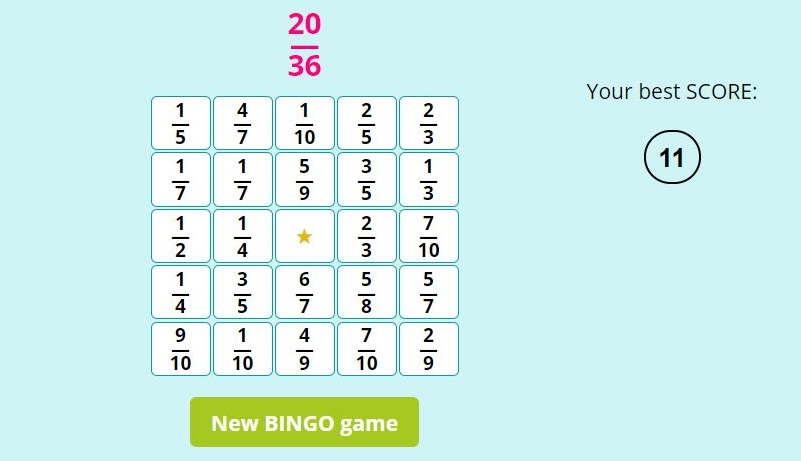Page No. 1096

# Simplifying fractions BINGO

Simplifying fractions BINGO online. Play simplifying fractions games online. simplifying fractions online game. Reducing fractions game online. fraction bingo game printable. online simplifying fractions game. math Bingo fractions game. simplifying fractions activity. bingo fractions math game. simplifying fractions online games. Fraction BINGO generator. reducing fractions online games. simplifying fractions interactive game. Online fraction BINGO free. Bingo fractions math game. bingo fractions math game. Printable simplify fractions games. Simplifying fraction BINGO printable free.

B I N G O

New BINGO game

Simplify the pink fraction and click on the correct answer in the table.

## Simplifying fractions BINGO games ONLINEThere is a fraction above the BINGO table. You must click on one fraction that is a simplified fraction of the given pink fraction. The correct answer can be always found in the fraction BINGO table. The object of this reducing fractions game is to get five stars in a column, row or diagonally.

## How to play simplifying fraction BINGO game?

Simplify the given fraction and look for the answer on the boxes and click on it. For example, we are looking for the result of simplifying fraction 20/36. The correct answer is 5/9, so click on the fraction 5/9 in the BINGO chart.

If your answer is correct, the number changes to a star , if your answer isn´t correct, the box will flash red and you have to click an another fraction.

The centre of the fraction BINGO table is already pre-filled with a star.### Simplifying fractions BINGO

You can see the SCORE of this simplifying fraction game on the right side. The SCORE means the number of clicks before you got BINGO. The lower the SCORE the better.

## Free simplifying fractions BINGO printables

Download these free printable simplifying fractions BINGO games. You can generate as many different simplifying fractions BINGO printables as you want.

This no-prep BINGO fractions game contains a grid of fractions which should be simplified. Instuctions are included. The back side contains correct answers of simplifying fractions.

## Free simplifying fractions worksheets PDF

Download these free printable simplifying fractions worksheets in PDF. You can generate as many different worksheets for simplifying fractions as you want. Find all our fractions worksheets here.

## What is simplifying fractions?

Simplifying fractions, also known as reducing fractions, is the process of expressing a fraction in its simplest or most reduced form. In this form, the numerator (the top number) and the denominator (the bottom number) have no common factors other than 1.

By dividing both parts of the fraction by the GCD, you effectively reduce the fraction to its simplest form.

The key concept behind simplifying fractions is to divide both the numerator and the denominator of the fraction by their greatest common divisor (GCD) or greatest common factor (GCF). The GCD is the largest number that can evenly divide both the numerator and the denominator without leaving a remainder.

The greatest common divisor (GCD) and greatest common factor (GCF) are the same thing.

### How do you simplify fractions?

Simplifying fractions is important in mathematics because it helps make calculations and comparisons easier and more intuitive. Here are the steps to simplify a fraction. For example, let's simplify the fraction 8/12:

2. Find the GCD of the numerator (8) and the denominator (12). The GCD of 8 and 12 is 4.
3. Divide both the numerator (8) and the denominator (12) by the GCD (4): (8 ÷ 4)/(12 ÷ 4) = 2/3
4. So, 8/12 simplifies to 2/3, which is its simplest form.

## Reducing fractions games

Our simplifying fractions games are fraction games designed to help students or individuals practice and improve their skills in reducing fractions.

## Simplifying fractions games

These simplifying fractions online games are math BINGO games which can be used in classrooms, tutoring sessions, or as part of online educational resources to make learning math concepts more engaging and interactive. This simplifying fractions BINGO is a fun way for anyone to hone their fraction simplification skills.

## Simplifying fractions online games

Simplifying fractions games are designed with an educational context in mind. Players are required to perform simplification steps to convert the fractions into their simplest form.

Our simplifying fractions games are interactive. The interactive nature makes learning more engaging and enjoyable. To motivate players to improve their simplification skills I add a competitive element: SCORE.

Category: